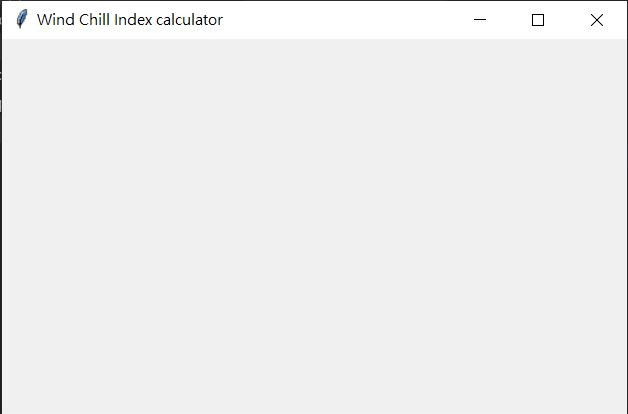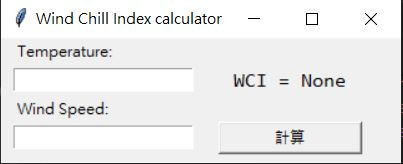#DAY 28
0

## Day28-Build python GUI using tkinter

``````import tkinter as tk

WindChillIndex = 0

win = tk.Tk()
win.title("Wind Chill Index calculator")

win.geometry('500x300')  # 這裡的乘是小x
win.mainloop()
``````
• `tk.TK()` 先宣告一個框架，就像話話你得先有一張紙一樣
• `.title()` 這個可以設定視窗的標題
• `.geometry` 則是設定視窗的大小
• `.mainloop()` 可以想像成一個常駐的 while 迴圈，是視窗程式很重要的部分，因為要一直監聽著各個按鈕是元件有沒有被使用者改變，這部分比較抽象，需要一點時間去思考。``````import tkinter as tk
import tkinter.font as tkFont

WindChillIndex = 0

#定義計算行為，這個我們明天再介紹
def calc(temp,wind):
pass

win = tk.Tk()
win.title("Wind Chill Index calculator")
win.geometry('320x100')
#設定標籤
tk.Label(win,text = "Temperature:").grid(column=0, row=0, sticky="W", padx=10)

tempVar = tk.StringVar()
tempEntry = tk.Entry(win, width=20, textvariable=tempVar).grid(column=0, row=1, padx=10)

#設定標籤
tk.Label(win,text = "Wind Speed:").grid(column=0, row=2, sticky="w", padx=10)

windVar = tk.StringVar()
windEntry = tk.Entry(win, width=20, textvariable=windVar).grid(column=0, row=3, padx=10)

ResultVar = tk.StringVar()
ResultVar.set("WCI = None")
ResultLabel = tk.Label(win,textvariable = ResultVar, font=tkFont.Font(family='consolas', size=12)).grid(column=1, row=1, padx=10)

b = tk.Button(win, text="計算",width=15, height=1, command=calc(temp,wind))
b.grid(column=1, row=3, padx=10)
win.mainloop()
``````

### grid()參數

• column 該元件位於的欄數
• row 該元件位於的列數
• sticky 該元件貼附的位置，有 N,E,S,W(北東南西)可以選擇
• padx 該元件與其他元件的水平間距
• pady 該元件與其他元件的垂直間距

Entry 上面多了一個 `var = tk.StringVar()` 這是在宣告 tk 的字串變數，tk 元件內用的變數不是一般的 str，所以要特別宣告成 tk.StringVar()。

.set() 是 tk.StringVar() 的寫入方法，相對的 .get() 則是他的讀取方式，也就是說 tk.StringVar() 的讀寫分別為下面這樣

``````TKstr = tk.StringVar()
TKstr.set("Hello") #寫
print(TKstr.get()) #讀
``````

Button 的宣告方法和其他物件相同，但他多了一個 `command=calc(temp,wind)` 而 calc(temp,wind) 有沒有很熟悉，我們在上面宣告過呢！因為這代表當這個按鈕被點擊時，就會去執行 calc(temp,wind)，詳細功能操作就明天再介紹囉~